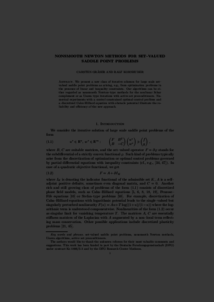Repository: Freie Universität Berlin, Math Department

# Nonsmooth Newton methods for set-valued saddle point problems

Gräser, C. and Kornhuber, R. (2009) Nonsmooth Newton methods for set-valued saddle point problems. SIAM Journal on Numerical Analysis, 47 (2). pp. 1251-1273. ISSN 0036-1429Preview

276kB

Official URL: http://dx.doi.org/10.1137/060671012

## Abstract

We present a new class of iterative schemes for large scale set-valued saddle point problems as arising, e.g., from optimization problems in the presence of linear and inequality constraints. Our algorithms can be regarded either as nonsmooth Newton-type methods for the nonlinear Schur complement or as Uzawa-type iterations with active set preconditioners. Numerical experiments with a control constrained optimal control problem and a discretized Cahn–Hilliard equation with obstacle potential illustrate the reliability and efficiency of the new approach.

Item Type: Article Set-valued saddle point problems, nonsmooth Newton methods, Uzawa algorithms, active set preconditioners Mathematical and Computer Sciences > Mathematics > Numerical Analysis Department of Mathematics and Computer Science > Institute of Mathematics 1802 Ekaterina Engel 19 Feb 2016 08:43 03 Mar 2017 14:41

Repository Staff Only: item control page